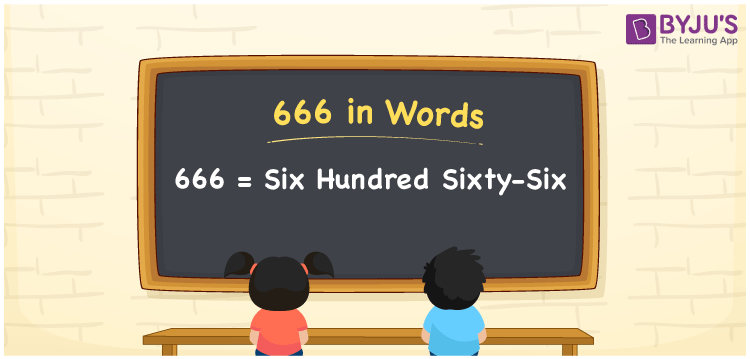# 666 in Words

666 in words can be written as Six Hundred Sixty-Six. The number name of 666 can be written using the place value chart. If you buy groceries for Rs. 666 from the store, then you can say that “I bought groceries for Six Hundred Sixty-Six Rupees from the store”. Numbers in words are curated by the faculty to help students ace the exams. 666 can be read as “Six Hundred Sixty-Six” in English.

 666 in words Six Hundred Sixty-Six Six Hundred Sixty-Six in Numbers 666

## 666 in English Words## How to Write 666 in Words?

Three digits are present in the number 666 and its place value can be found in the table which is given below.

 Hundreds Tens Ones 6 6 6

666 in expanded form can be written as:

6 × Hundred + 6 × Ten + 6 × One

= 6 x 100 + 6 x 10 + 6 x 1

= 600 + 60 + 6

= 666

= Six Hundred Sixty-Six

Hence, 666 in words is written as Six Hundred Sixty-Six.

666 is a natural number that precedes 667 and succeeds 665.

666 in words – Six Hundred Sixty-Six

Is 666 an odd number? – No

Is 666 an even number? – Yes

Is 666 a perfect square number? – No

Is 666 a perfect cube number? – No

Is 666 a prime number? – No

Is 666 a composite number? – Yes

## Frequently Asked Questions on 666 in Words

Q1

### How to write 666 in words?

666 can be written in words as “Six Hundred Sixty-Six”.
Q2

### Write Six Hundred Sixty-Six in numbers.

Six Hundred Sixty-Six in numbers can be written as 666.
Q3

### Is 666 an even number?

666 is an even number as it is completely divisible by 2.